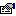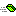## TripleDES

Represents the interface for Triple Data Encryption Standard classes to implement.

### Public:

#### Properties:

NameDescriptionBlockSize (get) Gets the block size, in bits, used by the cryptographic operation.BlockSize (let) Sets the block size, in bits, used by the cryptographic operation.FeedbackSize (get) Gets the feedback size, in bits, used by the cryptographic operation.FeedbackSize (let) Sets the feedback size, in bits, used by the cryptographic operation.IV (get) Gets the Initialization Vector used by the cryptographic operation.IV (let) Sets the Initialization Vector used by the cryptographic operation.Key (get) Returns the secret key used by the cryptographic operation.Key (let) Sets the secret key to be used by the cryptographic operation.KeySize (get) Returns the size of the key, in bits, used by the cryptographic operation.KeySize (let) Sets the size of the key, in bits.LegalBlockSizes (get) Gets a list of legal block sizes.LegalKeySizes (get) Gets a list of legal key sizes.Mode (get) Gets the mode the current algoritm is set to.Mode (let) Sets the mode the current algorithm is set to.Padding (get) Gets the padding to be used by the algorithm.Padding (let) Sets the padding to be used by the algorithm.

#### Methods:

NameDescriptionClear Releases all resources.CreateDecryptor Creates a new decryptor using the Triple Data Encryption Standard algorithm.CreateEncryptor Creates a new encryptor using the Triple Data Ecryption Standard algorithm.Equals Returns if this instance and the value are equal.GenerateIV Generates a new Initialization Vector array.GenerateKey Generates a new Key based on the key size.GetHashCode Returns a semi-unique value that represents this instance.ToString Returns a string representation of this instance.ValidKeySize Checks if the specific number of bits is a valid key size.